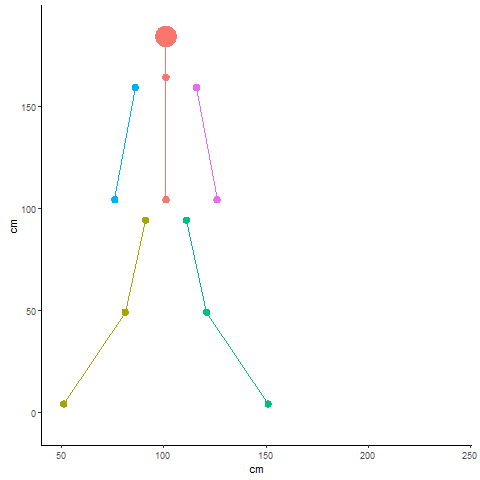# Animating ggforce::geom_circle() using gganimate gives bad animation. #157

Closed
opened this issue Aug 10, 2019 · 0 comments
Closed

# Animating ggforce::geom_circle() using gganimate gives bad animation.#157

opened this issue Aug 10, 2019 · 0 comments

###steenharsted commented Aug 10, 2019
 Hi, Thank you for your great packages gganimate and ggforce. I am having difficulties animating ggforce::geom_circle(). The geom_circle() objects are not properly animated. The animation seems to only include the raw data points. The animation of the standard geom_path() works as intended. ``````code: library(tidyverse) library(gganimate) library(ggforce) person <- tribble( ~object, ~x, ~y, ~group, ~size, "head", 100, 180, 1, 10, "neck", 100, 160, 1, 3, "pelvis", 100, 100, 1, 3, "lhip", 90, 90, 2, 3, "rhip", 110, 90, 3, 3, "lknee", 80, 45, 2, 3, "rknee", 120, 45, 3, 3, "lfoot", 50, 0, 2, 3, "rfoot", 150, 0, 3, 3, "lshoulder", 85, 155, 4, 3, "rshoulder", 115, 155, 5, 3, "lhand", 75, 100, 4, 3, "rhand", 125, 100, 5, 3) frame <- c(1, 10, 20, 30, 40, 50, 60, 70, 80, 90) data_for_plot <- crossing( frame, person) %>% mutate( x = x + frame, y = y + sin(frame)*5) plot_data <- ggplot(data_for_plot, aes(x0=x, y0=y, r=size/2, group=group, fill=factor(group), color=factor(group))) + geom_circle() + geom_path(aes(x,y)) + coord_equal() + labs(x="cm", y="cm") + theme_classic() + theme(legend.position = "none") plot_data+ transition_states(frame) ``````